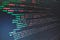# From XML to Pandas dataframesPhoto by Florian Olivo on Unsplash
`<data>    <student name="John">        <email>john@mail.com</email>        <grade>A</grade>        <age>16</age>    </student>    <student name="Alice">        <email>alice@mail.com</email>        <grade>B</grade>        <age>17</age>    </student>    <student name="Bob">        <email>bob@mail.com</email>        <grade>C</grade>        <age>16</age>    </student>    <student name="Hannah">        <email>hannah@mail.com</email>        <grade>A</grade>        <age>17</age>    </student></data>`
`import xml.etree.ElementTree as et xtree = et.parse("students.xml")xroot = xtree.getroot()`
`for node in xroot:     s_name = node.attrib.get("name")    s_mail = node.find("email").text    s_grade = node.find("grade").text    s_age = node.find("age").text`
`import pandas as pd import xml.etree.ElementTree as et xtree = et.parse("students.xml")xroot = xtree.getroot() df_cols = ["name", "email", "grade", "age"]rows = []for node in xroot:     s_name = node.attrib.get("name")    s_mail = node.find("email").text if node is not None else None    s_grade = node.find("grade").text if node is not None else None    s_age = node.find("age").text if node is not None else None        rows.append({"name": s_name, "email": s_mail,                  "grade": s_grade, "age": s_age})out_df = pd.DataFrame(rows, columns = df_cols)`
`import pandas as pdimport xml.etree.ElementTree as etdef parse_XML(xml_file, df_cols):     """Parse the input XML file and store the result in a pandas     DataFrame with the given columns.         The first element of df_cols is supposed to be the identifier     variable, which is an attribute of each node element in the     XML data; other features will be parsed from the text content     of each sub-element.     """        xtree = et.parse(xml_file)    xroot = xtree.getroot()    rows = []        for node in xroot:         res = []        res.append(node.attrib.get(df_cols))        for el in df_cols[1:]:             if node is not None and node.find(el) is not None:                res.append(node.find(el).text)            else:                 res.append(None)        rows.append({df_cols[i]: res[i]                      for i, _ in enumerate(df_cols)})        out_df = pd.DataFrame(rows, columns=df_cols)            return out_df`
`{df_cols[i]: res[i] for i, _ in enumerate(df_cols)}`

Written by

## Roberto Preste

#### I’m a PhD student in Bioinformatics, located in Bari (IT). I like writing about programming, data science and bioinformatics.

Welcome to a place where words matter. On Medium, smart voices and original ideas take center stage - with no ads in sight. Watch
Follow all the topics you care about, and we’ll deliver the best stories for you to your homepage and inbox. Explore
Get unlimited access to the best stories on Medium — and support writers while you’re at it. Just \$5/month. Upgrade